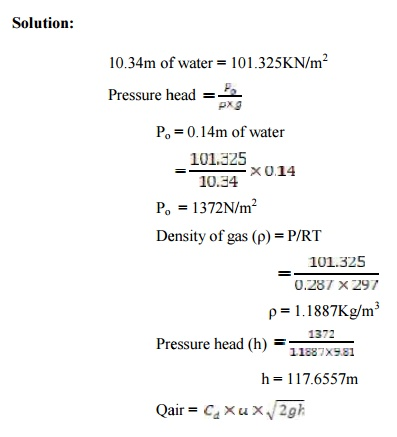Home | | Thermal Engineering I | Solved Problems: Internal Combustion Engines

# Solved Problems: Internal Combustion Engines

Mechanical and Electrical - Thermal Engineering - Internal Combustion Engines

Solved Problems:

1. A trial carried out in a four stroke single cylinder gas engine gave the following results. Cylinder dia=300 mm, Engine stroke=500mm, Clearance volume=6750cc, Explosions per minute=100  Pmax KN/m2    = 765 Net work load on the brake=190kg Brake dia=1.5m Rope dia=2 5mm, Speed of the engine=240rpm, Gas used=30 m3/kghr   , Calorific value of gas=2 0515 KJ/ m3 . Determine compression rat io, mechanical efficiency, indicated thermal efficiency, air standard efficiency, relative efficiency, assume r=1.4

GIVEN DATA:-

Dia of cylind er (d)=300mm=0.3m

Engine stroke(l)=500mm=0.5m

Clearance volume(vc)=6750/1003=6.75m3

Explosions pe r minute(n)=100/minute=i.67/sec

Pmin=765 KN /m2

Brake drum dia(D1)=1.5m

Rope dia(d1)= 0.025m

TO FIND:-

Compression rati o (r)

Mechanical efficiency mech)

Indicated thermal efficiency it)

Air standard efficiency (ηair)

Relative efficiency (ηrel)2. The following observat ions are recorded during a test on a four-stroke petrol engine, F.C = 3000 of fuel in 12sec, speed of the engine is 2500rpm, B.P = 20KW, Air intake orifice diameter = 35 mm,Pressure across the orifice = 140mm of water coefficient of discharge of orifice = 0.6 , piston diameter = 150mm, stroke length = 100 mm, Density of the fuel = 0.85gm/cc , r=6 .5, Cv of fuel = 42000KJ/Kg, Barometric pressure = 760mm of Hg , Room temperature = 24oc

Determine:

(i) Volumetric ef ficiency on the air basis alone

(ii)             Air-fuel ratio

(iii)           The brake mean effective pressure

(iv)           The relative efficiency on the brake thermal efficiency

Given data:

Fuel c onsumption = 30cc in 12sec

Speed (N) = 2500/60 rps

Brake power = 20KW

Orifice diameter (do) = 0.035 m

Pressure across the orifice (Po) = 140mm of water

Coefficient of discharge (Cd) = 0.6

Piston diameter (d) = 150mm = 0.15m Stroke length (l) = 0.1m

Density of fuel (ρ) = 0.85gm/ cc Compression ratio (r) = 6.5 Room temperature (Ta) = 297 K

Barometric pressure = 760mm of Hg = 101.325KK/m2= 10.34m of water To Find:

(i)               Volumet ric efficiency on the air basis alone

(ii)             Air-fuel ratio

(iii)          The brake mean effective pressure

(iv)          The relative efficiency on the brake thermal efficiency

Solution:Relative efficiency on brake thermal efficiency basis (ηrel) = ηBT/ ηair

= 0.22409/0.52703

ηrel = 42.52%

Study Material, Lecturing Notes, Assignment, Reference, Wiki description explanation, brief detail
Mechanical and Electrical : Thermal Engineering : Internal Combustion Engines : Solved Problems: Internal Combustion Engines |

Related Topics